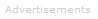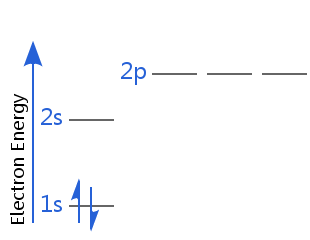# Definition of Electron Spin

## What is Electron Spin?

Electron spin is a quantum property of electrons. It is a form of angular momentum. The magnitude of this angular momentum is permanent. Like charge and rest mass, spin is a fundamental, unvarying property of the electron.As a teaching method, instructors sometimes liken electron spin to the earth spinning on its own axis every 24 hours. If the electron spins clockwise on its axis, it is described as spin-up; counterclockwise is spin-down. This is a convenient explanation, if not fully justifiable mathematically.

The spin angular momentum associated with electron spin is independent of orbital angular momentum, which is associated with the electron's journey around the nucleus.

Electron spin is not used to define electron shells, subshells, or orbitals, unlike the quantum numbers n, l, and ml.

## Electron Spin vs the Electron Spin Quantum Number

Electron spin and the electron spin quantum number are often treated as one and the same thing. Both are quantum numbers. They are in fact different, but very closely related concepts.

• electron spin has symbol s

• the electron spin quantum number has symbol ms.

### s

Electron spin, s, has only one possible value. That value is ½. Like the electron's rest mass and charge, spin does not vary.

### ms

Unlike the electron's mass and charge, spin has an orientation. The spin's orientation is shown by the spin quantum number, ms.

ms can be positive or negative.

Different ways of expressing ms are to write:

+   or   −

spin-up   or   spin-downorFor example, in the diagram below helium is in its electron ground state.

One of its electrons is spin-up, the other is spin-down. Alternatively, one of its electrons has positive spin, the other has negative spin.It is usual to say that any electron in an atom is fully characterized by four quantum numbers:

n: the principal quantum number

l: the orbital angular momentum quantum number

ml: the magnetic quantum number

ms: the spin quantum number

We do not include s, because its value does not vary. It is always one-half. So the value of the spin quantum number, ms, is generally written as ±½.

## The Discovery of Electron Spin

The electron's built-in or intrinsic spin was first detected by Otto Stern and Walther Gerlach in 1922. However, Erwin Schrödinger's wave equation of 1926 did not account for it.

Wolfgang Pauli successfully introduced electron spin into wave mechanics as an add-on. Three quantum numbers were already in use; Pauli's introduction of spin completed the quantum picture of the electron in a hydrogen atom.

In 1928, Paul Dirac's famous relativistic equation for the electron, the Dirac Equation, produced electron spin as a natural consequence of quantum physics: spin was no longer an add-on.Search the Dictionary for More Terms